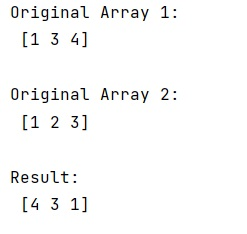# Sort array's rows by another array

Learn, how to sort array's rows by another array in Python?
Submitted by Pranit Sharma, on January 22, 2023

NumPy is an abbreviated form of Numerical Python. It is used for different types of scientific operations in python. Numpy is a vast library in python which is used for almost every kind of scientific or mathematical operation. It is itself an array which is a collection of various methods and functions for processing the arrays.

## Sorting array's rows by another array

Suppose that we are given two NumPy arrays (arr1 and arr2) and we need to sort arr1 in descending order, and to have the current relationship between arr1 and arr2 to be maintained (i.e., after sorting both, the rows of arr1 and arr2[0, :] are the same).

For this purpose, we will use numpy.argsort() method. First, we will use argsort() on arr1 and store this result in some variables. We will then sort the arr1 by the reverse of the sorted arr1. Similarly, we will sort the arr2 by the reverse of sorted arr1.

Let us understand with the help of an example,

## Python code to sort array's rows by another array

```# Import numpy
import numpy as np

# Creating a numpy array
arr1 = np.array([1,3,4])

# Display original array 1
print("Original Array 1:\n",arr1,"\n")

# Create another array
arr2 = np.array([1,2,3])

# Display original array 2
print("Original Array 2:\n",arr2,"\n")

# Sorting the array
arr1_s = arr1.argsort()
sorted_arr1 = arr1[arr1_s[::-1]]

# Display result
print("Result:\n",sorted_arr1,"\n")
```

Output:What's New (MCQs)

Top Interview Coding Problems/Challenges!

IncludeHelp's Blogs

Languages: » C » C++ » C++ STL » Java » Data Structure » C#.Net » Android » Kotlin » SQL
Web Technologies: » PHP » Python » JavaScript » CSS » Ajax » Node.js » Web programming/HTML
Solved programs: » C » C++ » DS » Java » C#
Aptitude que. & ans.: » C » C++ » Java » DBMS
Interview que. & ans.: » C » Embedded C » Java » SEO » HR
CS Subjects: » CS Basics » O.S. » Networks » DBMS » Embedded Systems » Cloud Computing
» Machine learning » CS Organizations » Linux » DOS
More: » Articles » Puzzles » News/Updates